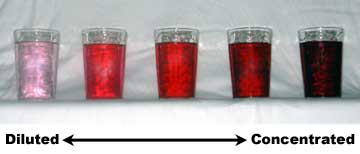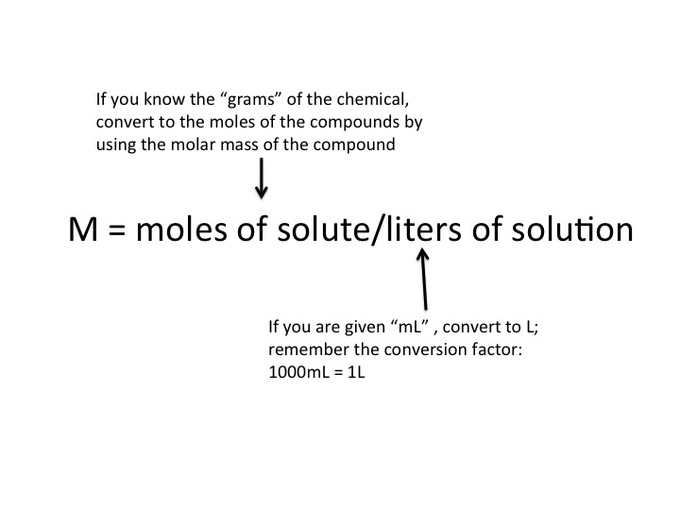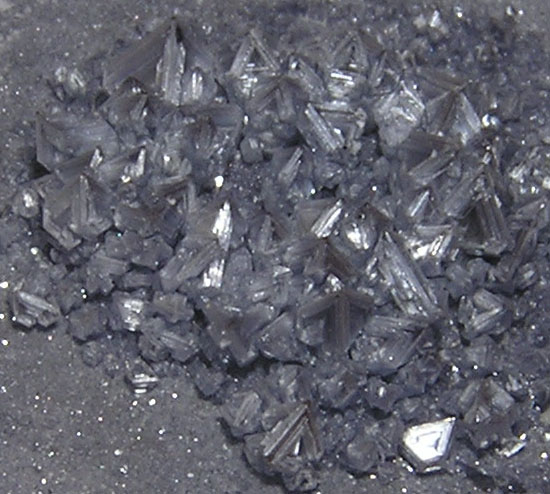Dilutions of Solutions

Diluting a solution involves adding additional solvent to decrease the solution’s concentration.

Learning Objectives

Calculate the concentration of a diluted solution.

Key Takeaways

Key Points

• Most commonly, a solution ‘s concentration is expressed in terms of mass percent, mole fraction, molarity, molality, and normality. When calculating dilution factors, it is important that the units of volume and concentration remain consistent.
• Dilution calculations can be performed using the formula M1V1 = M2V2.
• A serial dilution is a series of stepwise dilutions, where the dilution factor is held constant at each step.

Key Terms

• dilution: a solution that has had additional solvent, such as water, added to make it less concentrated
• serial dilution: stepwise dilution of a substance in solution

Dilution refers to the process of adding additional solvent to a solution to decrease its concentration. This process keeps the amount of solute constant, but increases the total amount of solution, thereby decreasing its final concentration. Dilution can also be achieved by mixing a solution of higher concentration with an identical solution of lesser concentration. Diluting solutions is a necessary process in the laboratory, as stock solutions are often purchased and stored in very concentrated forms. For the solutions to be usable in the lab (for a titration, for instance), they must be accurately diluted to a known, lesser concentration.

The volume of solvent needed to prepare the desired concentration of a new, diluted solution can be calculated mathematically. The relationship is as follows:

$\text{M}_1\text{V}_1=\text{M}_2\text{V}_2$

M1 denotes the concentration of the original solution, and V1 denotes the volume of the original solution; M2 represents the concentration of the diluted solution, and V2 represents the final volume of the diluted solution. When calculating dilution factors, it is important that the units for both volume and concentration are the same for both sides of the equation.

Example

• 175 mL of a 1.6 M aqueous solution of LiCl is diluted with water to a final volume of 1.0 L. What is the final concentration of the diluted solution?
• $\text{M}_1\text{V}_1=\text{M}_2\text{V}_2$
• (1.6 M)(175 mL) = M2(1000 mL)
• M2 = 0.28 MDilutions: Dilutions can sometimes be visually observed. In the image above, the intense red color slowly fades as the solutions become more diluted.

Serial Dilutions

Serial dilutions involve diluting a stock or standard solution multiple times in a row. Typically, the dilution factor remains constant for each dilution, resulting in an exponential decrease in concentration. For example, a ten-fold serial dilution could result in the following concentrations: 1 M, 0.1 M, 0.01 M, 0.001 M, and so on. As is evidenced in this example, the concentration is reduced by a factor of ten in each step. Serial dilutions are used to accurately create extremely diluted solutions, as well as solutions for experiments that require a concentration curve with an exponential or logarithmic scale. Serial dilutions are widely used in experimental sciences, including biochemistry, pharmacology, microbiology, and physics.

Solving Dilution Problems in Solution Chemistry CLEAR & SIMPLE – YouTube: This video shows how to solve two dilution problems, using the standard dilution formula, M1V1 = M2V2.

Using Molarity in Calculations of Solutions

Molarity is a unit of concentration; it is equal to moles of solute divided by the total volume of the solution in liters.

Learning Objectives

Translate between molarity, grams of solute in solution, and volume of solution.

Key Takeaways

Key Points

• Molar concentration, also called molarity, is the number of moles of solute per liter of solution. Molarity is the most common measurement of solution concentration.
• Because molarity measurements are mole/L measurements, we often use this unit for stoichiometric calculations to determine the amount of chemical in a given mixture.
• Do not confuse moles with molarity: molarity is a measure of concentration, while moles are a measure of the amount of substance.

Key Terms

• molarity: The concentration of a substance in solution, expressed as the number moles of solute per liter of solution.
• solution: a homogeneous mixture, either liquid, gas, or solid, formed by dissolving one or more substances
• mole:

Molarity

In chemistry, molar concentration, or molarity, is defined as moles of solute per total liters of solution. This is an important distinction; the volume in the definition of molarity refers to the volume of the solution, and not the volume of the solvent. The reason for this is because one liter of solution usually contains either slightly more or slightly less than 1 liter of solvent, due to the presence of the solute. The SI unit for molarity is is mol/m3; however, you will almost always encounter molarity with the units of mol/L. A solution of concentration 1 mol/L is also denoted as “1 molar” (1 M). Mol/L can also be written in the following ways (however, mol/L, or simply M, is most common):

1 mol/L = 1 M = 1 mol/dm3 = 1 mol dm−3 = 1000 mol/m3

It is important to distinguish moles from molarity; molarity is a measurement of concentration while moles are a measure of the amount of substance present at a given time.Molarity: Molarity is a measurement of concentration, with units of mol solute per liter solution.

Using Molarity in Calculations Involving Solutions

Molarity can be used in a various calculations involving solutions. The following formula is very useful, as it relates the molarity of the solution, the total volume of the solution (in liters), and the number of moles solute:

$\text{MV}=\text{mol}$

Example 1

A student pipettes a 100 mL sample of a 1.5 M solution of potassium bromide. How many moles of potassium bromide are contained in the sample?

$\text{MV}=\text{mol}$

(1.5 M)(0.100 L) = mol

mol = 0.15 mol KBr

Notice in the example above that volume must be converted to L from mL.

You might notice that the above formula bears some resemblance to our dilution formula:

$\text{M}_1\text{V}_1=\text{M}_2\text{V}_2$

Because we now know that MV = mol, we can simplify our the dilution formula to the following:

$\text{mol}_1=\text{mol}_2$

This shouldn’t surprise us. After all, in any dilution, what changes is the amount of solvent, while the number of moles of solute remains constant throughout.

Example 2

What is the molarity of a solution containing 0.32 moles of NaCl in 3.4 liters of solution?

$\text{M}=\frac{0.32\text{ mol NaCl}}{3.4\text{ L solution}}={0.094\text{ M NaCl}}$

Molarity Practice Problems – YouTube: This video demonstrates practice problems with molarity, calculating the moles and liters to find the molar concentration. It also uses conversion factors to convert between grams and moles and between milliliters and liters.

Molarity Practice Problems (Part 2) – YouTube: Use molarity to convert between mass and volume in a solution. This video looks at how to use molarity as a conversion factor. If you know the molarity, you can solve for either the number of moles or the volume of a solution. Also, molarity is a ratio that describes the moles of solute per liter of solution.

Solution Stoichiometry

Stoichiometry can be used to calculate the quantitative relationships between species in aqueous solution.

Learning Objectives

Calculate concentrations of solutions in molarity, molality, mole fraction and percent by mass and volume.

Key Takeaways

Key Points

• Stoichiometry deals with the relative quantities of reactants and products in chemical reactions. It can be used to find the quantities of the products from given reactants in a balanced chemical reaction, as well as percent yield.
• To calculate the quantity of a product, calculate the number of moles for each reactant. Moles of a product are equal to the moles of a limiting reactant in one-to-one reaction stoichiometry. To find product mass, moles must be multiplied by the product’s molecular weight.
• In stoichiometric calculations involving solutions, a given solution’s concentration is often used as a conversion factor.

Key Terms

• stoichiometry: the study and calculation of quantitative (measurable) relationships of the reactants and products in chemical reactions (chemical equations)
• molarity: the concentration of a substance in solution, expressed as the number moles of solute per liter of solution
• molality: the concentration of a substance in solution, expressed as the number of moles of solute per kilogram of solvent

Concentration of Solutions

Recall that a solution consists of two components: solute (the dissolved material) and solvent (the liquid in which the solute is dissolved). The amount of solute in a given amount of solution or solvent is known as the concentration. The two most common ways of expressing concentration are molarity and molality.

Molarity

The molar concentration (M) of a solution is defined as the number of moles of solute (n) per liter of solution (i.e, the volume, Vsolution):

$\text{M}=\frac{\text{n}}{\text{V}_{\text{solution}}}$

The units of molarity are mol/L, often abbreviated as M.

For example, the number of moles of NaCl in 0.123L of a 1.00M solution of NaCl can be calculated as follows:

$0.123\text{ L of solution} \times \frac{1.00 \text{ mole}}{1.00 \text{ L of solution}} = 0.123 \text { moles NaCl}$

Molality

The molal concentration (m) of a solution is defined as the number of moles of solute (n) per kilogram of solvent (i.e., the mass of the solvent, msolvent):

$\text{m}=\frac{\text{n}}{\text{m}_{\text{solvent}}}$

The units of molality are mol/kg, or m.

For example, the number of moles of NaCl dissolved in 0.123kg of H2O (the solvent), in order to make a 1.00m solution of NaCl, can be calculated as follows:

$0.123 \text{ kg of solvent} \times \frac{1.00 \text{ mole}}{1.00 \text{ kg of solvent}} = 0.123 \text { moles NaCl}$

Reaction Stoichiometry in Solutions

We can perform stoichiometric calculations for aqueous phase reactions just as we can for reactions in solid, liquid, or gas phases. Almost always, we will use the concentrations of the solutions as conversion factors in our calculations.

Example

• 123 mL of a 1.00 M solution of NaCl is mixed with 72.5 mL of a 2.71 M solution of AgNO3. What is the mass of AgCl(s) formed in the precipitation reaction?Crystals of silver chloride (AgCl): Stoichiometry deals with the relative quantities of reactants and products in chemical reactions. It can be used to find the quantities of the products from given reactants in a balanced chemical reaction, as well as percent yield.

First, we need to write out our balanced reaction equation:

$\text{AgNO}_3(aq)+\text{NaCl}(aq)\rightarrow\text{AgCl}(s)+\text{NaNO}_3(aq)$

The next step, as in any calculation involving stoichiometry, is to determine our limiting reactant. We can do this by converting both of our reactants into moles:

$\text{123 mL NaCl}\times \frac{\text{1 L}}{\text{1000 mL}} \times \frac{\text{1.00 mol NaCl}}{\text{1 L}}= \text{0.123 mol NaCl}$

$\text{72.5 mL AgNO}_3 \times \frac{\text{1 L}}{\text{1000 mL}} \times \frac{\text{2.71 mol AgNO}_3}{\text{1 L}}= \text{0.196 mol AgNO}_3$

We can see from our reaction equation that AgNO3 and NaCl react in a 1:1 ratio. Because there are fewer moles of NaCl present in solution, NaCl is our limiting reactant. We can now solve for the mass of AgCl formed:

$\text{123 mL NaCl} \times \frac{\text{1 L}}{\text{1000 mL}} \times \frac{\text{1.00 mol NaCl}}{\text{1 L}} \times \frac {\text{1 mol AgCl}}{\text{1 mol NaCl}} \times \frac {\text{143 g}}{\text{1 mol AgCl}}=\text{17.6 g AgCl}$

Therefore, 17.6 g AgCl(s) is formed in the reaction.

To sum up: we converted to each reactant’s moles by using the given concentrations as conversion factors, expressing molarity as mol/L; once we found our limiting reactant, we converted through to grams of AgCl formed.